Log in Register FAQ Calendar Search Today's Posts Mark Forums Read Contact Us Archive styles# Welcome to the MacNN Forums.If this is your first visit, be sure to check out the FAQ by clicking the link above. You may have to register before you can post: click the register link above to proceed. To start viewing messages, select the forum that you want to visit from the selection below.You are here: MacNN Forums > Software - Troubleshooting and Discussion > Developer Center > Demo Crypto ProblemDemo Crypto ProblemGhoser777 Professional Poster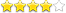Join Date: Dec 2000 Location: Chicago, Illinois Status: Offline May 20, 2004, 09:42 PM I was hoping to show my class some sample code I had written in java demonstrating how public private key encryption works. I thought I got the mathematics implemented correctly (it actually does work sometimes), but something seems to be missing. Can anyone spot what's wrong with my algorithm? ```Code: import java.math.*; import java.util.*; public class CryptoInfo { private BigInteger n; //public mod private BigInteger e; //public key private BigInteger d; //private key public static void main(String[] args) { String message = "Hello World!"; CryptoInfo me = new CryptoInfo(new BigInteger("5"), new BigInteger("19")); CryptoInfo you = new CryptoInfo(new BigInteger("23"), new BigInteger("29")); String encryptMess = me.prepareSignedMessage(message, you.getPublicEncryptionKey(), you.getPublicModulus()); System.out.println(encryptMess); String decryptMess = you.readSignedMessage(encryptMess, me.getPublicEncryptionKey(), me.getPublicModulus()); System.out.println(decryptMess); } //should make sure p and q are valid primes public CryptoInfo(BigInteger p, BigInteger q) { n = p.multiply(q); //phi(n) equals this only because p and q are distinct primes BigInteger phiN = p.subtract(BigInteger.ONE).multiply(q.subtract(BigInteger.ONE)); e = randomEncryptionKey(phiN); d = e.modInverse(phiN); } public BigInteger getPublicEncryptionKey() { return e; } public BigInteger getPublicModulus() { return n; } //prepares a signed, encrypted message for the user with passed in public key and public mod public String prepareSignedMessage(String message, BigInteger otherKey, BigInteger otherMod) { BigInteger[] big = encrypt(bigIntsForMessage(message), d, n); //encode using our private key big = encrypt(big, otherKey, otherMod); //encode using their public key return stringRepForBigInts(big); } //decodes a signed message from the user with the passed in public key and public mod public String readSignedMessage(String encryptedMessage, BigInteger otherKey, BigInteger otherMod) { BigInteger[] big = encrypt(bigIntsForStringRep(encryptedMessage), d, n); //decode our using private key big = encrypt(big, otherKey, otherMod); //decode using their public key System.out.println(stringRepForBigInts(big)); return messageForBigInts(big); } //returns a BigInteger g between 2 and phiN-1 such that //g and phiN are relatively prime private BigInteger randomEncryptionKey(BigInteger phiN) { BigDecimal r; BigInteger g; BigDecimal decPhi = new BigDecimal(phiN); do { r = new BigDecimal(Math.random()); r = r.multiply(decPhi); g = r.toBigInteger(); } while(g.compareTo(BigInteger.ONE) <= 0 || !g.gcd(phiN).equals(BigInteger.ONE)); //do this whil g is less than or equal to 1 or g is not relatively prime to phiN return g; } //encrypts an array of BigInteger values using the passed in key and modulus public BigInteger[] encrypt(BigInteger[] code, BigInteger key, BigInteger mod) { BigInteger[] array = new BigInteger[code.length]; for(int i=0; ibeamso Fresh-Faced Recruit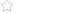Join Date: Nov 2001 Location: Melboune, Australia Status: Offline May 21, 2004, 02:36 AM Just so I understand what you're doing, you're signing the message before encrypting the message for the other party to decrypt. I can't work out your encryption function, but try using BigInteger.modPow() rather the code you've got there ( I don't understand what it's doing). This is just a pedantic thing, but try and encrypt single numbers rather than arrays of numbers.Ghoser777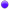Professional PosterJoin Date: Dec 2000 Location: Chicago, Illinois Status: Offline May 21, 2004, 06:33 AM modPow gives me the exact same (wrong) answer - I was just calculating the modPow manually before. So strange... Thanks, Matt FahrenbacherGhoser777Professional PosterJoin Date: Dec 2000 Location: Chicago, Illinois Status: Offline May 21, 2004, 07:50 AM Okay... perhaps it's my random encrpytion key that is messed up? I think that method does what I want (e and phiN are relatively prime by the end), but it seems that based on which random encryption key is chosen, sometimes I get an encoding/decoding scheme that works, other times I don't. Weird.... Matt FahrenbacherGhoser777Professional PosterJoin Date: Dec 2000 Location: Chicago, Illinois Status: Offline May 22, 2004, 07:33 PM Okay, I get what the problem is now... I just don't know a good fix for it. If person A has public mod of 30 and person B has public mod of 21, then the scenario is something like this: encrypt(M, key, 30) (value will be between 0 and 29) encrypt(M, key, 21) (takes a value from 0 to 29 and converts it to a value from 0 to 20) encrypt(M, key, 21) (takes a value from 0 to 20 and converts it to a value from 0 to 20) encrypt(M, key, 30) (takes a value from 0 to 20 and converts it to a value from 0 to 29) Now here's where things get ugly - the middle two operations are suppose to undo each other - so after them, we should be back to where we were after only on encrypt call. Unfortunately, if the first call to encrypt leaves us with an int between 21 and 29 (possible), then the next two calls of encrypt will not return us back to where we want to be. Instead we will end up with a value between 0 and 20... which is why I keep getting garbage after the final encrypt call. Okay, now that I've isolated the problem (I hope), does anyone have an idea of how to solve it? Thanks, Matt FahrenbacherGhoser777Professional PosterJoin Date: Dec 2000 Location: Chicago, Illinois Status: Offline May 23, 2004, 02:30 PM Fixed! Well, the comments indicate that there still could be a potential bug everyone in a while, but it should be very very very rare. Thanks everyone for letting me express my problem (look at the readSignedMessage method for the comments)! Matt Fahrenbacher ```Code: import java.math.*; import java.util.*; public class CryptoInfo { private BigInteger n; //public mod private BigInteger e; //public key private BigInteger d; //private key private Random r = new Random(); public static void main(String[] args) { CryptoInfo me = new CryptoInfo(); CryptoInfo you = new CryptoInfo(); String message = "Is this working yet?!?!?!"; BigInteger yourPublicKey = you.getPublicEncryptionKey(); BigInteger yourPublicMod = you.getPublicModulus(); BigInteger myPublicKey = me.getPublicEncryptionKey(); BigInteger myPublicMod = me.getPublicModulus(); String encryptMess = me.prepareSignedMessage(message, yourPublicKey, yourPublicMod); String decryptMess = you.readSignedMessage(encryptMess, myPublicKey, myPublicMod); System.out.println(decryptMess); } //Creates a CryptoInfo instance using primes of size 10 bits public CryptoInfo() { this(10); } //Creates a CryptoInfo instance using primes with the indicated total number of bits public CryptoInfo(int bits) { if(bits <= 6) throw new IllegalArgumentException("Bits must exceed 6"); BigInteger nth, mth; //need nth and mth to be distinct numbers so we're not picking p and w to be the same prime //nth and mth refer to which prime we're considering, so we want to at least pick a prime that is three digits long //so we need to pick a number that is at least the 26th prime do nth = new BigInteger(bits, r); while(nth.compareTo(new BigInteger("26"))<0); do mth = new BigInteger(bits, r); while(mth.compareTo(new BigInteger("26"))<0 || mth.equals(nth)); initialize(nthPrime(nth),nthPrime(mth)); } //creates a CryptoInfo instance using the indicated two primes public CryptoInfo(BigInteger p, BigInteger q) { initialize(p,q); } //private helper method needed because you can only call other constructors //on the first line of a constructor private void initialize(BigInteger p, BigInteger q) { if(!isPrime(p) || !isPrime(q)) throw new IllegalArgumentException("Either p or q (or both) are not prime"); else if(p.equals(q)) throw new IllegalArgumentException("p and q are not distinct primes"); n = p.multiply(q); //phi(n) equals this only because p and q are distinct primes BigInteger phiN = p.subtract(BigInteger.ONE).multiply(q.subtract(BigInteger.ONE)); System.out.println("P:" + p + "\nQ:" + q + "\nN:" + n + "\nphiN:" + phiN); e = randomEncryptionKey(phiN); d = e.modInverse(phiN); } //returns the public encryption key for the current CryptoInfo public BigInteger getPublicEncryptionKey() { return e; } //returns the public modulus for the current CryptoInfo public BigInteger getPublicModulus() { return n; } //returns the private encryption key for the current CryptoInfo private BigInteger getPrivateKey() { return d; } //prepares a signed, encrypted message for the user with passed in public key and public mod public String prepareSignedMessage(String message, BigInteger otherKey, BigInteger otherMod) { BigInteger[] big = encrypt(bigIntsForMessage(message), getPrivateKey(), getPublicModulus()); //encode using our private key big = encrypt(big, otherKey, otherMod); //encode using their public key return stringRepForBigInts(big); } //decodes a signed message from the user with the passed in public key and public mod public String readSignedMessage(String encryptedMessage, BigInteger otherKey, BigInteger otherMod) { BigInteger[] big = encrypt(bigIntsForStringRep(encryptedMessage), getPrivateKey(), getPublicModulus()); //decode using our private key BigInteger[] temp = encrypt(big, otherKey, otherMod); //decode using their public key //In the temp array, we might end up with values that make no sense because of this reason: //Let's say my public mod is 30 and yours is 21. //If when I encrypt using my private key I get a value between 22 and 29, then encrypting //with your public key and then with your private key will at most give me a value in the //range of 0 to 20. But to correctly get back to where we were, we need to end up getting //a value between 22 and 29 (which is impossible). So to fix this, in the event that we //get a decrypted value that isn't a valud ascii value, we need to add to the pre-decrypted //value the proper modulus enough times tell after we decrypt, we get something useful. //i.e. //Say we encrypt the letter 'c' and we get 28 mod 30 //Then when we encrypt and decrypt 28 mod 21, we get the number 7 //But the middle stage of our encryption should just undo each //other and we should get 28 back. So instead, we need to add //21 to our answer to get 28 back. (Because 28 % 21 == 7... so we //lost some info) //In theory, this could break in the event where we wrap around so many times //that we end up at a number between 0 and 127. But this will (hopefully) be //rare for our uses. BigInteger max = new BigInteger("127"); for(int i=0; i 0) //descending, tempInt is bigger than max { big[i] = big[i].add(getPublicModulus()); temp[i] = encrypt(big[i], otherKey, otherMod); } } return messageForBigInts(temp); } //returns a BigInteger g between 2 and phiN-1 such that //g and phiN are relatively prime private BigInteger randomEncryptionKey(BigInteger phiN) { BigDecimal r; BigInteger g; BigDecimal decPhi = new BigDecimal(phiN); do { r = new BigDecimal(Math.random()); r = r.multiply(decPhi); g = r.toBigInteger(); } while(g.compareTo(BigInteger.ONE) <= 0 || !g.gcd(phiN).equals(BigInteger.ONE)); //do this whil g is less than or equal to 1 or g is not relatively prime to phiN return g; } //encrypts an array of BigInteger values using the passed in key and modulus public BigInteger[] encrypt(BigInteger[] code, BigInteger key, BigInteger mod) { BigInteger[] array = new BigInteger[code.length]; for(int i=0; ibeamso Fresh-Faced RecruitJoin Date: Nov 2001 Location: Melboune, Australia Status: Offline May 24, 2004, 08:45 PM Originally posted by Ghoser777: Okay, I get what the problem is now... I just don't know a good fix for it. If person A has public mod of 30 and person B has public mod of 21, then the scenario is something like this: encrypt(M, key, 30) (value will be between 0 and 29) encrypt(M, key, 21) (takes a value from 0 to 29 and converts it to a value from 0 to 20) encrypt(M, key, 21) (takes a value from 0 to 20 and converts it to a value from 0 to 20) encrypt(M, key, 30) (takes a value from 0 to 20 and converts it to a value from 0 to 29) Now here's where things get ugly - the middle two operations are suppose to undo each other - so after them, we should be back to where we were after only on encrypt call. Unfortunately, if the first call to encrypt leaves us with an int between 21 and 29 (possible), then the next two calls of encrypt will not return us back to where we want to be. Instead we will end up with a value between 0 and 20... which is why I keep getting garbage after the final encrypt call. Okay, now that I've isolated the problem (I hope), does anyone have an idea of how to solve it? Okay... I was told this one in class last night. The steps you outline above are signing with the sender's private key and then encrypting with the recipient's public key. As the recipient's public key is smaller than the senders, the input may undergo a modulus operation prior to the exponentiation under modulus operation. This can result in the incorrect decryption of the message. What you can do in the above case is perform the operations in reverse, ie. encrypt then sign. Code your algorithm to detect this case (in encryption and decryption) and there shouldn't be any problems with the decryption returning unexpected results.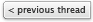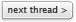Thread ToolsShow Printable VersionEmail this PageYou are here: MacNN Forums > Software - Troubleshooting and Discussion > Developer Center > Demo Crypto Problem Public Forums:Forum RulesYou may not post new threads You may not post replies You may not post attachments You may not edit your posts BB code is On Smilies are On [IMG] code is On HTML code is OffTop Privacy Policy All times are GMT -4. The time now is 09:07 PM. All contents of these forums © 1995-2017 MacNN. All rights reserved. Branding + Design: www.gesamtbild.com vBulletin v.3.8.8 © 2000-2017, Jelsoft Enterprises Ltd.,